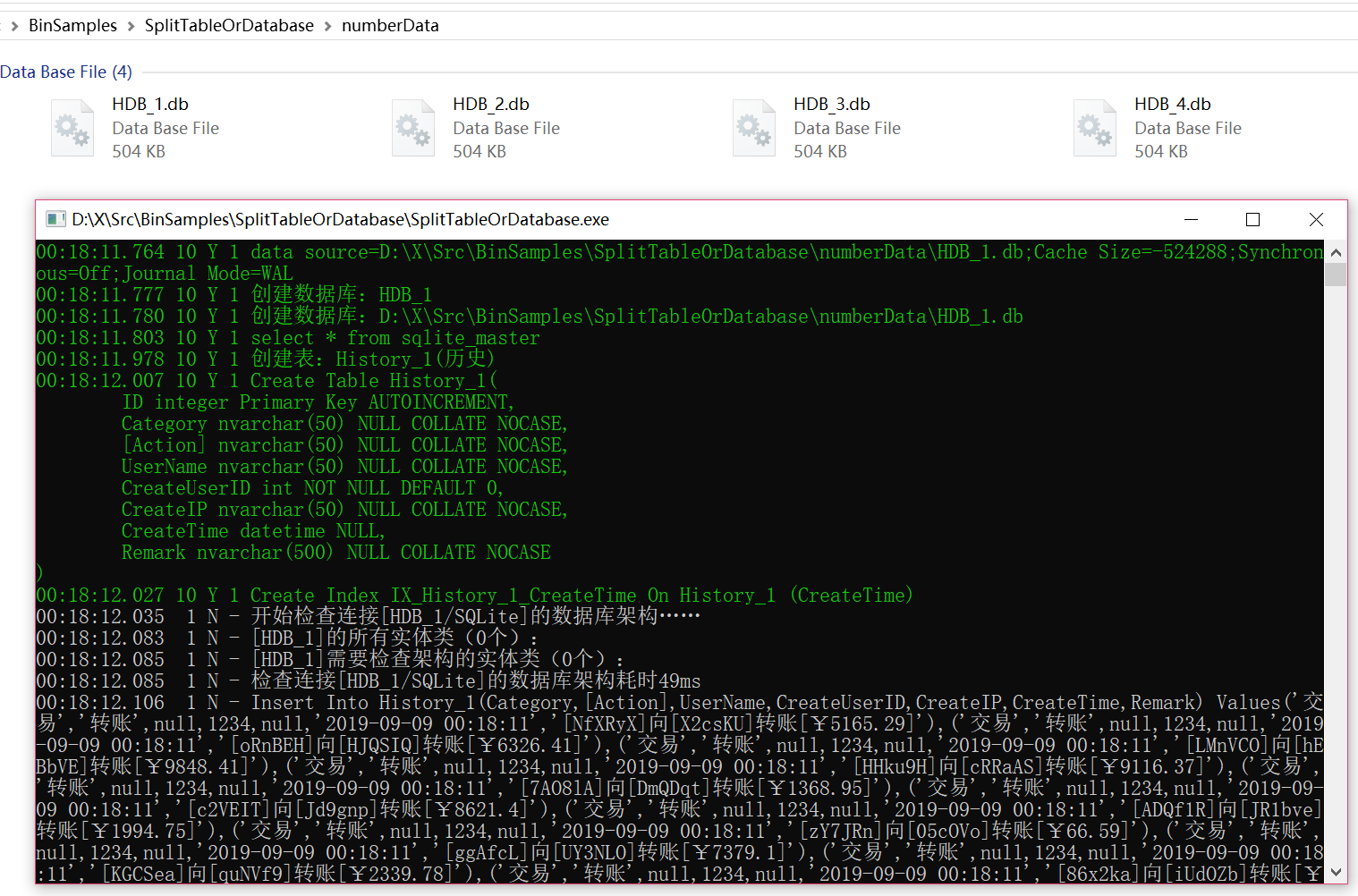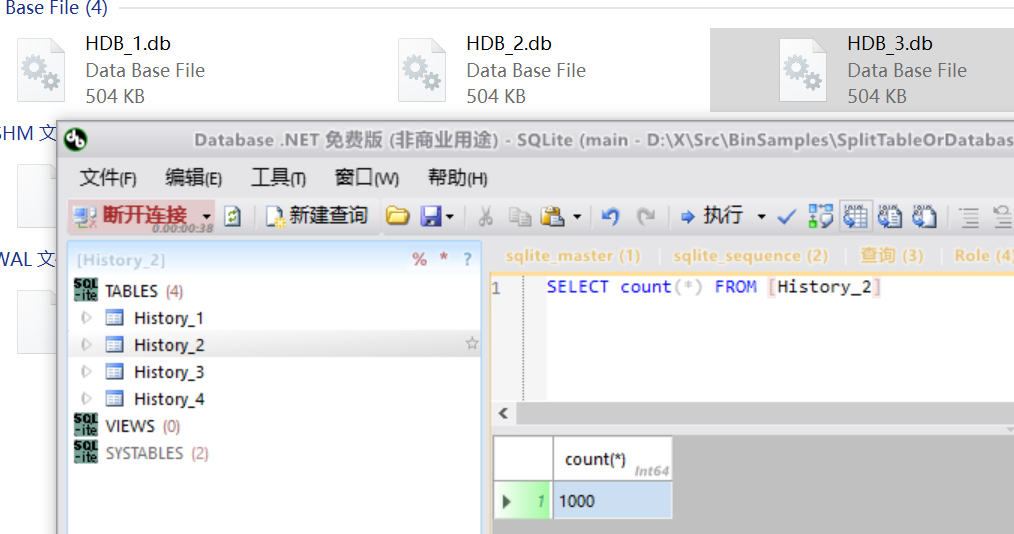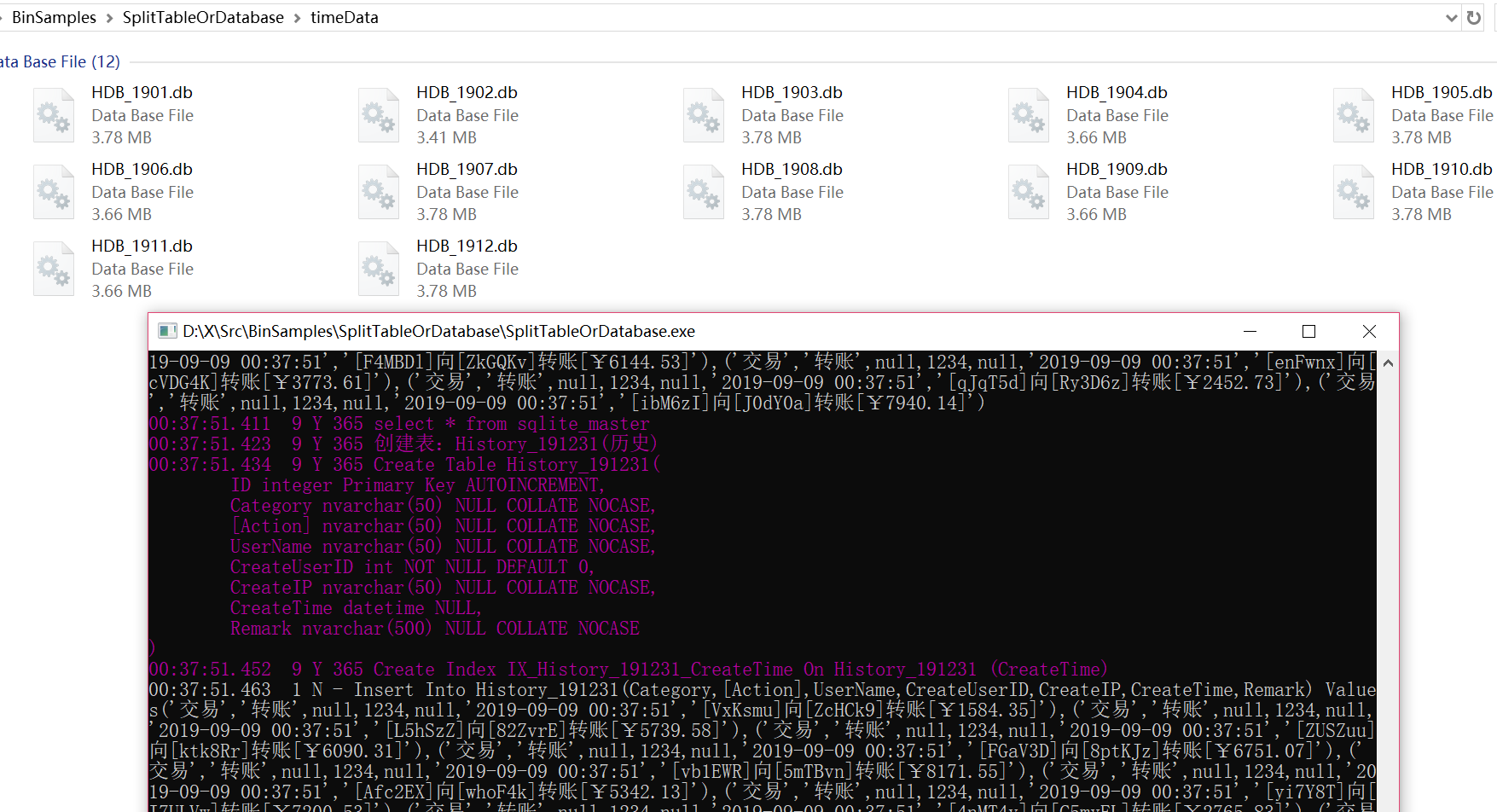NewLife.XCode是一个有15年历史的开源数据中间件，支持netcore/net45/net40，由新生命团队(2002~2019)开发完成并维护至今，以下简称XCode。

XCode是重度充血模型，以单表操作为核心，不支持多表关联Join，复杂查询只能在where上做文章，整个select语句一定是from单表，因此对分表操作具有天然优势！

！！ 阅读本文之前，建议回顾《百亿级性能（索引的威力）》，其中“索引完备”章节详细描述了大型数据表的核心要点。

100亿数据其实并不多，一个比较常见的数据分表分库模型：

MySql数据库8主8从，每服务器8个库，每个库16张表，共1024张表（从库也有1024张表） ，每张表1000万到5000万数据，整好100亿到500亿数据！

# 全自动分表

```static SampleData()
{
// 配置自动分表策略，一般在实体类静态构造函数中配置
Meta.ShardPolicy = new TimeShardPolicy(nameof(Id), Meta.Factory)
{
ConnPolicy = "{0}",
TablePolicy = "{0}_{1:yyyyMMdd}",
Step = TimeSpan.FromDays(1),
};
}```

1. 添加。从主键雪花Id中解析得到时间，再根据时间策略计算得到表名，生成Insert语句；
2. 删除。从主键雪花Id中解析得到时间，再根据时间策略计算得到表名，生成Delete语句；
3. 修改。从雪花Id得知时间，结合策略得到表名，生成Update语句；
4. 单查。从雪花Id得知时间，结合策略得到表名，生成Select查询语句；
5. 批查。必须带有时间范围，并且使用WhereExpression表达式，否则直接抛出异常。从表达式中找到时间区间，结合策略得到多个表名，依次执行查询，直到满足分页行数为止；

```public static IList<DeviceData> Search(Int32 deviceId, String topics, DateTime start, DateTime end, String key, PageParameter page)
{
var exp = new WhereExpression();

if (deviceId >= 0) exp &= _.DeviceId == deviceId;
if (!topics.IsNullOrEmpty()) exp &= _.Topic.In(topics.Split(","));
exp &= _.Id.Between(start, end, Meta.Factory.Snow);
if (!key.IsNullOrEmpty()) exp &= _.Topic.Contains(key) | _.Data.Contains(key) | _.Creator.Contains(key) | _.CreateIP.Contains(key);

return FindAll(exp, page);
}```

Tips:全自动用主键字段分表时，不能启用自增

`<Column Name="ID" DataType="Int64" PrimaryKey="True" Description="编号" />`

```// 分表分库
Meta.ShardPolicy = new TimeShardPolicy(nameof(ID), Meta.Factory)
{
//ConnPolicy = "{0}",
TablePolicy = "{0}_{1:yyyyMMdd}",
Step = TimeSpan.FromDays(1),
Field = _.ID
};```

```        /// <summary>搜索数据集</summary>
/// <param name="p"></param>
/// <returns></returns>
protected virtual IEnumerable<TEntity> Search(Pager p)
{
var start = p["dtStart"];
var end = p["dtEnd"];
var act = Request.Path.ToUriComponent();
var key = p["q"];
var filterSos = p["filterSos"];

var whereExpression = Entity<TEntity>.SearchWhereByKeys(key);

#region 时间查询
var findTime = Entity<TEntity>.Meta.Factory.AllFields.Find(x =>
{
if (act.IndexOf("User") == -1)
{
return x.Field.ColumnName.Equals("CreateTime");
}
else
{
return x.Field.ColumnName.Equals("RegisterTime");
}
});
if (findTime == null)
findTime = Entity<TEntity>.Meta.Factory.MasterTime;

if (findTime != null)
{
var startTime = start != null ? start.ToDateTime().ToString("yyyy-MM-dd 00:00:00").ToDateTime(): DateTime.Now.Date;
var endTime = end != null ? end.ToDateTime(): DateTime.Now;

var shardPolicy = Entity<TEntity>.Meta.ShardPolicy as TimeShardPolicy;
if (shardPolicy != null)
{
if(shardPolicy.Field!=null)
whereExpression &= shardPolicy.Field.Between(startTime, endTime, Entity<TEntity>.Meta.Factory.Snow);
else
whereExpression &= Entity<TEntity>.Meta.Factory.Unique.Between(startTime, endTime, Entity<TEntity>.Meta.Factory.Snow);
}
else
whereExpression &= findTime.Between(startTime, endTime);
}
#endregion

// 根据模型列设置，拼接作为搜索字段的字段
var modelTable = ModelTable;
var modelCols = modelTable?.GetColumns()?.Where(w => w.ShowInSearch)?.ToList() ?? new List<ModelColumn>();

foreach (var col in modelCols)
{
var val = p[col.Name];
if (val.IsNullOrWhiteSpace()) continue;
var fields = Entity<TEntity>.Meta.Table.FindByName(col.Name);
if (!(fields is null)) whereExpression &= fields == val;
}

//添加映射字段查询
foreach (var item in Entity<TEntity>.Meta.Factory.Fields)
{
var val = p[item.Name];
if (!val.IsNullOrWhiteSpace())
{
whereExpression &= item.Equal(val);
}
}

return Entity<TEntity>.FindAll(whereExpression, p);
}```

# 半自动分表

```public static IList<SampleData> Search(Int64 dataId, String traceId, DateTime start, DateTime end, PageParameter page)
{
var exp = new WhereExpression();

if (dataId > 0) exp &= _.DataId == dataId;
if (!traceId.IsNullOrEmpty()) exp &= _.TraceId == traceId;

// 时间区间倒序，为了从后往前查
return Meta.AutoShard(end.AddSeconds(1), start, () => FindAll(exp, page))
.FirstOrDefault(e => e.Count > 0) ?? new List<SampleData>();
}```

# 手工分表

```public static IList<TraceData> Search(Int32 appId, Int32 itemId, String clientId, String name, String kind, Int32 minError, DateTime start,DateTime end, String key, PageParameter page)
{
var exp = new WhereExpression();

if (appId >= 0) exp &= _.AppId == appId;
if (itemId > 0) exp &= _.ItemId == itemId;
if (!clientId.IsNullOrEmpty()) exp &= _.ClientId == clientId;
if (!name.IsNullOrEmpty()) exp &= _.Name == name;
if (!key.IsNullOrEmpty()) exp &= _.ClientId == key | _.Name == key;
if (minError > 0) exp &= _.Errors >= minError;

if (appId > 0 && start.Year > 2000)
{
var fi = kind switch
{
"day" => _.StatDate,
"hour" => _.StatHour,
"minute" => _.StatMinute,
_ => _.StatDate,
};

if (start == end)
exp &= fi == start;
else
exp &= fi.Between(start, end);
}
else
exp &= _.Id.Between(start, end, Meta.Factory.Snow);

using var split = Meta.CreateShard(start);

return FindAll(exp, page);
}```

# 分表原理

```History.Meta.ConnName = \$"HDB_{i + 1}";
History.Meta.TableName = \$"History_{j + 1}";```

```History.Meta.ConnName = \$"HDB_{i + 1}";
History.Meta.TableName = \$"History_{j + 1}";
try{
// todo 业务代码
}finally{
History.Meta.ConnName = null;
History.Meta.TableName = null;
}```

```using var split = History.Meta.CreateSplit(\$"HDB_{i + 1}", \$"History_{j + 1}");
// todo 业务代码```

# 例程剖析

```<Tables Version="9.12.7136.19046" NameSpace="STOD.Entity" ConnName="STOD" Output="" BaseClass="Entity" xmlns:xs="http://www.w3.org/2001/XMLSchema-instance" xs:schemaLocation="http://www.newlifex.com https://raw.githubusercontent.com/NewLifeX/X/master/XCode/ModelSchema.xsd" xmlns="http://www.newlifex.com/ModelSchema.xsd">
<Table Name="History" Description="历史">
<Columns>
<Column Name="ID" DataType="Int32" Identity="True" PrimaryKey="True" Description="编号" />
<Column Name="Category" DataType="String" Description="类别" />
<Column Name="Action" DataType="String" Description="操作" />
<Column Name="CreateUserID" DataType="Int32" Description="用户编号" />
<Column Name="CreateIP" DataType="String" Description="IP地址" />
<Column Name="CreateTime" DataType="DateTime" Description="时间" />
<Column Name="Remark" DataType="String" Length="500" Description="详细信息" />
</Columns>
<Indexes>
<Index Columns="CreateTime" />
</Indexes>
</Table>
</Tables>```

## 按数字散列分表分库

```static void TestByNumber()
{
XTrace.WriteLine("按数字分表分库");

// 预先准备好各个库的连接字符串，动态增加，也可以在配置文件写好
for (var i = 0; i < 4; i++)
{
var connName = \$"HDB_{i + 1}";
History.Meta.ConnName = connName;

// 每库建立4张表。这一步不是必须的，首次读写数据时也会创建
//for (var j = 0; j < 4; j++)
//{
//    History.Meta.TableName = \$"History_{j + 1}";

//    // 初始化数据表
//    History.Meta.Session.InitData();
//}
}

//!!! 写入数据测试

// 4个库
for (var i = 0; i < 4; i++)
{
var connName = \$"HDB_{i + 1}";
History.Meta.ConnName = connName;

// 每库4张表
for (var j = 0; j < 4; j++)
{
History.Meta.TableName = \$"History_{j + 1}";

// 插入一批数据
var list = new List<History>();
for (var n = 0; n < 1000; n++)
{
var entity = new History
{
Category = "交易",
Action = "转账",
CreateUserID = 1234,
CreateTime = DateTime.Now,
Remark = \$"[{Rand.NextString(6)}]向[{Rand.NextString(6)}]转账[￥{Rand.Next(1_000_000) / 100d}]"
};

}

// 批量插入。两种写法等价
//list.BatchInsert();
list.Insert(true);

// 恢复默认
History.Meta.TableName = null;
}
// 恢复默认
History.Meta.ConnName = null;
}
}```

```var connName = \$"HDB_{i + 1}";

```var connName = \$"HDB_{i + 1}";
History.Meta.ConnName = connName;
History.Meta.TableName = \$"History_{j + 1}";```## 按时间序列分表分库

```static void TestByDate()
{
XTrace.WriteLine("按时间分表分库，每月一个库，每天一张表");

// 预先准备好各个库的连接字符串，动态增加，也可以在配置文件写好
var start = DateTime.Today;
for (var i = 0; i < 12; i++)
{
var dt = new DateTime(start.Year, i + 1, 1);
var connName = \$"HDB_{dt:yyMM}";
}

// 每月一个库，每天一张表
start = new DateTime(start.Year, 1, 1);
for (var i = 0; i < 365; i++)
{
History.Meta.ConnName = \$"HDB_{dt:yyMM}";
History.Meta.TableName = \$"History_{dt:yyMMdd}";

// 插入一批数据
var list = new List<History>();
for (var n = 0; n < 1000; n++)
{
var entity = new History
{
Category = "交易",
Action = "转账",
CreateUserID = 1234,
CreateTime = DateTime.Now,
Remark = \$"[{Rand.NextString(6)}]向[{Rand.NextString(6)}]转账[￥{Rand.Next(1_000_000) / 100d}]"
};

}

// 批量插入。两种写法等价
//list.BatchInsert();
list.Insert(true);
}
}```例程遍历了今年的365天，在连接字符串指定的timeData目录下，生成了12个月份数据库，然后每个库里面按月生成数据表，每张表插入1000行模拟数据。

# 分表查询

```static void SearchByDate()
{
// 预先准备好各个库的连接字符串，动态增加，也可以在配置文件写好
var start = DateTime.Today;
for (var i = 0; i < 12; i++)
{
var dt = new DateTime(start.Year, i + 1, 1);
var connName = \$"HDB_{dt:yyMM}";
}

// 随机日期。批量操作
start = new DateTime(start.Year, 1, 1);
{
XTrace.WriteLine("查询日期：{0}", dt);

History.Meta.ConnName = \$"HDB_{dt:yyMM}";
History.Meta.TableName = \$"History_{dt:yyMMdd}";

var list = History.FindAll();
XTrace.WriteLine("数据：{0}", list.Count);
}

// 随机日期。个例操作
start = new DateTime(start.Year, 1, 1);
{
XTrace.WriteLine("查询日期：{0}", dt);
var list = History.Meta.ProcessWithSplit(
\$"HDB_{dt:yyMM}",
\$"History_{dt:yyMMdd}",
() => History.FindAll());

XTrace.WriteLine("数据：{0}", list.Count);
}
}```# 分表策略

• Oracle适合单表1000万~1亿行数据，要做分区
• MySql适合单表1000万~5000万行数据，很少人用MySql分区

• 日志型时间序列表，如果每月数据不足1000万，则按月分表，否则按天分表。缺点是数据热点极为明显，适合热表、冷表、归档表的梯队架构，优点是批量写入和抽取性能显著；
• 状态表（订单、用户等），按Crc16哈希分表，以1000万为准，决定分表数量，向上取整为2的指数倍（为了好算）。数据冷热均匀，利于单行查询更新，缺点是不利于批量写入和抽取；
• 混合分表。订单表可以根据单号Crc16哈希分表，便于单行查找更新，作为宽表拥有各种明细字段，同时还可以基于订单时间建立一套时间序列表，作为冗余，只存储单号等必要字段。这样就解决了又要主键分表，又要按时间维度查询的问题。缺点就是订单数据需要写两份，当然，时间序列表只需要插入单号，其它更新操作不涉及。

# 分表与分区对比

• 分区除了能建立子索引外，还可以建立全局索引，而分表不能建立全局索引；
• 分区能跨区查询，但非常非常慢，一不小心就扫描所有分区；
• 分表架构，很容易做成分库，支持轻易扩展到多台服务器上去，分区只能要求数据库服务器更强更大；
• 分区主要由DBA操作，分表主要由程序员控制；

！！！某项目使用XCode分表功能，已经过生产环境三年半考验，日均新增4000万~5000万数据量，2亿多次添删改，总数据量数百亿。